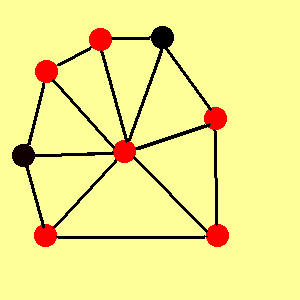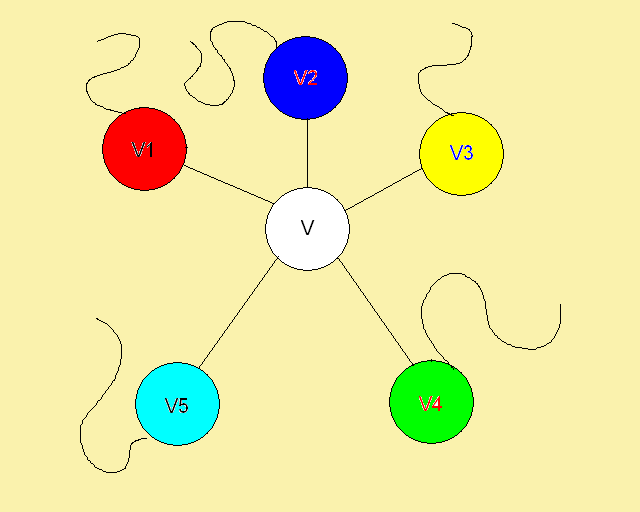Theorems

Euler’s Formula – Let G be a connected plane graph with n vertices, m edges, and l faces.  Then n-m+l = 2.ex. n = 8 vertices, m = 14 edges, l = 8 faces

n-m+l = 8-14+8 = 2 ڤ

Corollary – A plane graph with n ≥ 3 vertices has at most 3n-6 edges.

* From this we get: d(G) = 2m/n ≤ 2(3n - 6)/n < 6 *

4-color theorem – Every planar graph is 4-colorable.

5-color theorem – Every planar graph is 5-colorable.

6-color theorem – Every planar graph is 6-colorable.

The 6-color theorem proof

Proof by induction.

Trivial with planar graph with at most 6 vertices.  We can easily produce a 6 coloring with one color for each vertex.

Now, suppose that G is simple planar with n vertices, and that all simple planar graphs with n-1 vertices are 6 colorable.

G must contain a vertex v of degree at most 5.

If we delete v and it’s incident edges, the remaining graph has (n-1) edges and is thus 6-colorable.

A 6-coloring of G is obtained by coloring v with a different color from the (at most 5) vertices adjacent to v.  ڤ6-Coloring Greedy Algorithm

1. Sort the vertices according to Smallest Vertex Ordering.

2. For i = 1,...n.

• Let j be the smallest color not used in Pred(vi)

• Color vi with color j.

Complexity Analysis

We can sort the vertices (step 1) in O(nlogn) time and the loop takes O(n) time giving us an overall complexity of O(n).

PREVIOUS: Definitions    NEXT: 5-Color Theorem